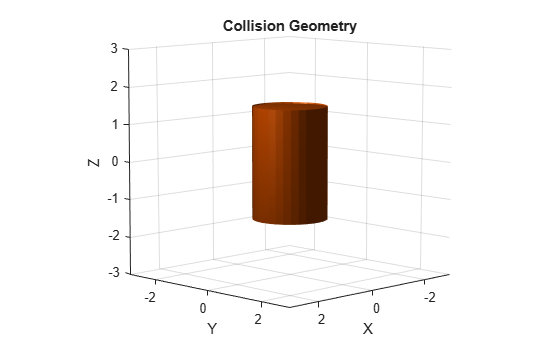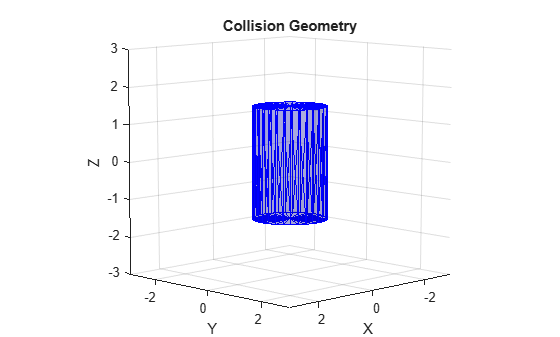# show

Show collision geometry

Since R2019b

## Syntax

``show(geom)``
``show(geom,"Parent",AX)``
``ax = show(___)``
``[ax,patchobj] = show(___)``

## Description

````show(geom)` shows the collision geometry in the current figure at its current pose. The function automatically generates the tessellation.```
````show(geom,"Parent",AX)` specifies the axes `AX` in which to plot the collision geometry.```

example

````ax = show(___)` returns the axes on which you plot the collision geometry.```
````[ax,patchobj] = show(___)` returns the graphic object `patchobj` that represents the collision geometry in the plot.```

## Examples

collapse all

Create a cylinder collision geometry. The cylinder has a length of 3 meters and a radius of 1 meter.

`cyl = collisionCylinder(1,3);`

Show the cylinder.

`show(cyl)`Show the cylinder in a new figure, and return the patch object that represents the cylinder. Change the cylinder color to cyan by changing the RGB value of the `FaceColor` field in the patch object. Hide the edges by setting `EdgeColor` to `'none'`.

```figure [~,patchObj] = show(cyl); patchObj.FaceColor = [0 1 1]; patchObj.EdgeColor = 'none';```## Input Arguments

collapse all

Collision geometry to show, specified as one of these objects:

Axes on which to plot the collision geometry, specified as an `Axes` object.

## Output Arguments

collapse all

Axes displaying the collision geometry, returned as an `Axes` object. For more information, see Axes Properties.

Graphic object that represents the collision geometry, returned as a `Patch` object. For more information, see Patch Properties.

## Version History

Introduced in R2019b